This mathematics ClipArt gallery offers 14 images of angles that are often used in trigonometry. Images include angles in all four quadrants, positive and negative angles, reference angles, and angles of depression and elevation.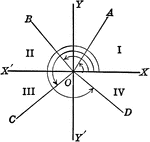Axes showing trigonometric angles, and quadrants.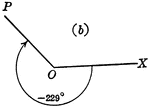### -229 Degree Trigonometric Angle

Trigonometric angle -229 degrees.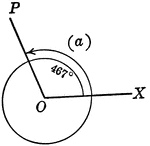### 467 Degree Trigonometric Angle

Trigonometric angle 467 degrees.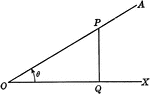### Acute Angle Theta

Illustration of acute angle theta as part of a right triangle.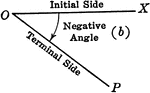### Negative Trigonometric Angle

Negative trigonometric angle with initial and terminal sides labeled.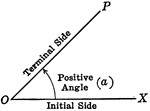### Positive Trigonometric Angle

Positive trigonometric angle with initial and terminal sides labeled.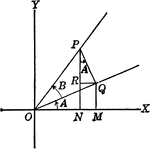### Angles Used to Illustrate Sum and Difference of Two Angles

Angles used to illustrate the sum and difference of two angles and trig identities.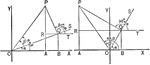### Sum of 2 Acute Angles

Illustration of two triangles, showing the sine of the sum of two acute angles expressed in terms of…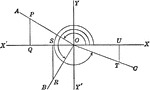### Reference Angles/Triangles Formed by Angles in Quadrants

Lines drawn to horizontal to form triangle ratios (reference triangles, angles).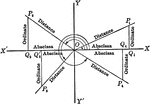### Reference Angles/Triangles Formed by Angles in Quadrants With Labels

Lines drawn to horizontal to form triangle ratios (reference triangles, angles). Axes, quadrants, abscissa,…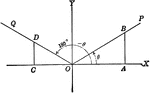### Coordinate Axis With Angles, Lines, and Perpendiculars Drawn

Coordinate axis with angle XOP equal to theta, Θ, and angle XOQ=180 - Θ. From any point in the terminal…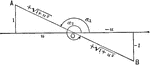### Negative Cotangent Angles

Illustration that can be used to show that if the cotangent of an angle is negative the angle must terminate…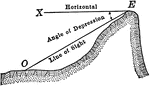### Angle of Depression

Illustration showing an angle of depression from a horizontal line to a line of sight.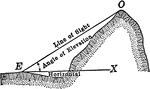### Angle of Elevation

Illustration showing an angle of elevation from a horizontal line to a line of sight.